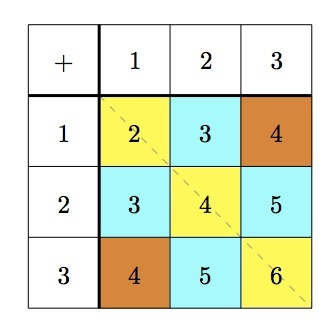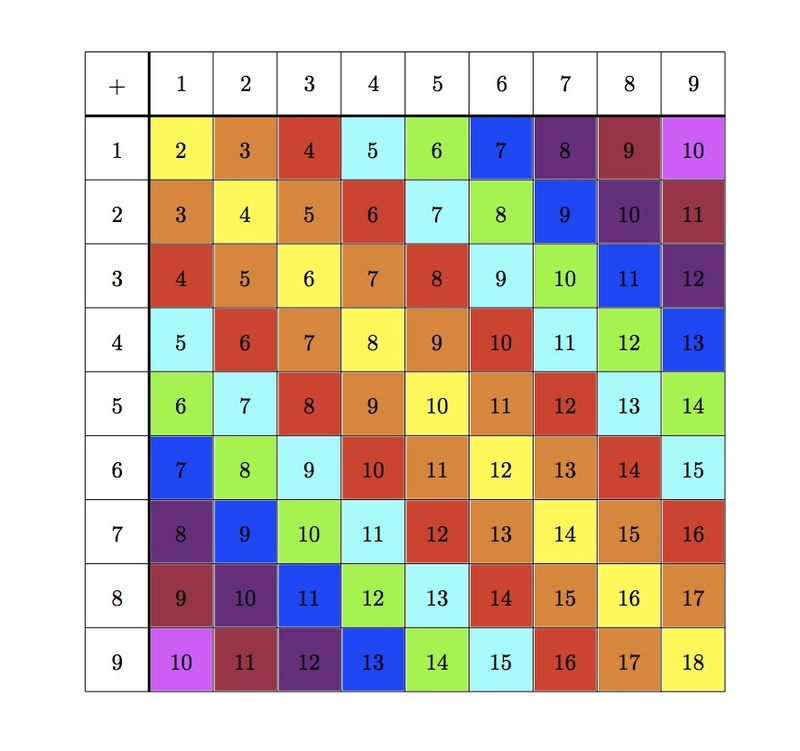# Symmetry of the addition table

Alignments to Content Standards: 3.OA.D.9

Below is a table showing how to add numbers from $1$ to $3$:Cut out the table and fold it over the dotted line. Notice that the blue squares match up and so do the orange squares. Notice that the squares that match up have the same numbers in them. We say that the squares that match up when you fold along the line are "mirror images" of each other.

The table below shows how to add numbers from $1$ to $9$. Two squares are shaded blue and two are green:1. Are the blue squares mirror images of each other? Explain why the numbers in the blue squares are equal.

2. Are the green squares mirror images of each other? Explain why the numbers in the green squares are equal.
3. Shade the rest of the mirror image squares with the same color. Why are the mirror image numbers always equal?

## IM Commentary

The goal of this task is to help students understand the commutative property of addition by examining the addition facts for single digit numbers. This is important as it gives students a chance, at a young age, to do more than memorize these arithmetic facts which they will use throughout their education. The approach taken here is a geometric one, associating the commutative property of addition with the symmetry of the addition table. Students don't "officially" study symmetry until 4th grade (see 4.G.3), so this task defines the particular symmetry for them.

Two additional interesting aspects of the table worth noting are:

• The first nine even numbers appear on the shaded diagonal. This comes from the fact, for example, that $4+4 = 2 \times 4$ and so gives students a chance to think about the meaning of multiplication. This is discussed for a smaller addition table in the task "Addition Patterns'' but the teacher might also prompt the students to address this here.
• The symmetry of the table about the diagonal essentially cuts in half the number of facts which the students need to memorize if they think flexibly about addition of single digit numbers.

This task is mainly intended for instructional purposes. There will be ample opportunities to test whether or not the students have learned their single digit addition tables and the goal of this task is to facilitate the process by revealing patterns and structure in the table.

## Solution

1. The two blue squares are mirror images of one another. One of them is two squares below the yellow $4$ and the other is two squares to the right of the yellow $4$: when the table is folded along the diagonal containing the yellow $4$, the two blue squares match up.

The two blue squares contain the sums $5 + 2$ and $2 + 5$. These are both equal to seven. They are the same because the same two numbers are being added: only the order is different. The commutative property of addition says that the sum does not depend on the order of the two numbers.

2. The two green squares are mirror images of one another. One of them is four squares below the yellow $8$ and the other is four squares to the right of the yellow $8$: when the table is folded along the diagonal containing the yellow $8$, the two green squares match up.

The two green squares contain the sums $8 + 4$ and $4 + 8$. These are both equal to twelve. They are the same because the same two numbers are being added: only the order is different. The commutative property of addition says that the sum does not depend on the order of the two numbers.

3. The table below has been shaded with mirror image squares being the same color. Squares next to the diagonal yellow squares are shaded orange, those two squares removed from the diagonal yellow squares are shaded red, and so on.The reason why mirror image squares have the same numbers is the commutative property of addition: the sum of two numbers is the same regardless of the order in which they are added. For example, the lower green diagonal of squares has the sums $5+1$, $6+2$, $7+3$, $8+4$, and $9+5$ while the upper green diagonal has the same sums in reverse order: $1+5$, $2+6$, $3+7$, $4+8$, and $5+9$. Since the order of the addends does not influence the sum, it is sufficient to know one of these diagonals and this then determines the other. So the commutative property of addition cuts the amount of facts that need to be memorized almost in half.